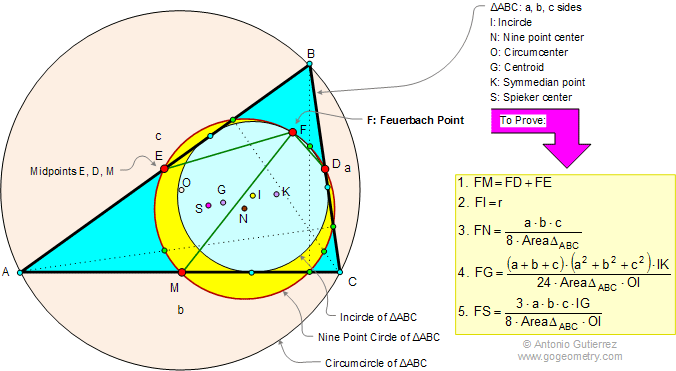Feuerbach Point Distances: Illustration The Feuerbach Point of a triangle ABC is the point where the incircle touches the nine-point circle circle of ABC. The figure below show the following distances: 1. Distance between the Feuerbach point F and the midpoints M, E, and D of the sides. 2. Distance between the Feuerbach point F and the incenter I. 3. Distance between the Feuerbach point F and the Nine point center N. 4. Distance between the Feuerbach point F and the centroid G. 5. Distance between the Feuerbach point F and the Spieker center S. See also: circumcenter, symmedian or Lemoine point, and Feuerbach theorem.Home | Triangle Centers | Feuerbach Index | Acute Triangle | Obtuse | Right| Nine Point Circle | Email | By Antonio Gutierrez